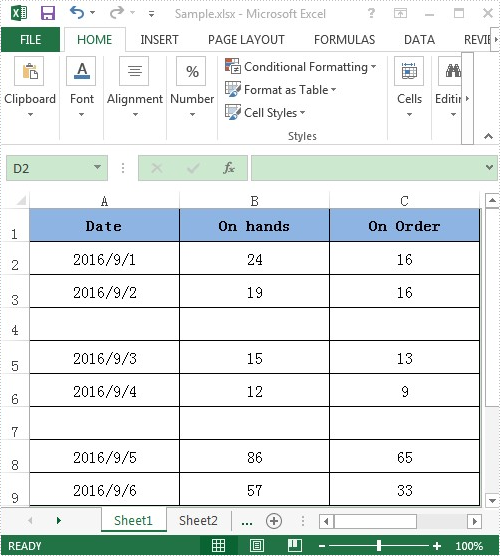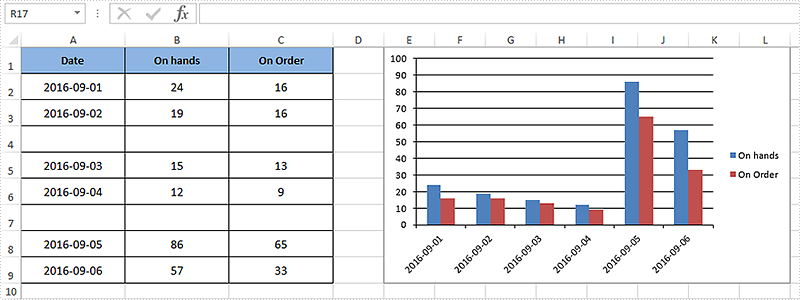# Use Discontinuous Data Range to Create Chart in Excel

Apart from creating chart with continuous data range, Spire.XLS also supports to create chart with discontinuous data range by calling the XlsRange.AddCombinedRange(CellRange cr) method. This example explains a quick solution of how to achieve this task in C# with the help of Spire.XLS.

For demonstration, here we used a template excel document, in which you can see there are some blank rows among the data, in other words, the data range is discontinuous.Here comes to the detail steps:

Step 1: Instantiate a Wordbook object, load the excel document and get its first worksheet.

```Workbook book = new Workbook();
Worksheet sheet = book.Worksheets;
```

Step 2: Add a column chart to the first worksheet and set the position of the chart.

```Chart chart = sheet.Charts.Add(ExcelChartType.ColumnClustered);
chart.SeriesDataFromRange = false;
//Set chart position
chart.LeftColumn = 5;
chart.TopRow = 1;
chart.RightColumn = 13;
chart.BottomRow = 10;
```

Step 3: Add two series to the chart, set data source for category labels and values of the series with discontinuous data range.

```//Add the first series
//Set name of the serie
cs1.Name = sheet.Range["B1"].Value;
//Set data source for Category Labels and Values of the serie with discontinuous data range
//Specify the serie type
cs1.SerieType = ExcelChartType.ColumnClustered;

cs2.Name = sheet.Range["C1"].Value;
cs2.SerieType = ExcelChartType.ColumnClustered;
```

Step 4: Save the excel document.

```book.SaveToFile("Result.xlsx", FileFormat.Version2010);
```

After executing the above example code, a column chart with discontinuous data range was added to the worksheet as shown below.Full code:

```using Spire.Xls;
using Spire.Xls.Charts;

namespace Assign_discontinuous_range_for_chart
{
class Program
{
static void Main(string[] args)
{
Workbook book = new Workbook();
Worksheet sheet = book.Worksheets;

chart.SeriesDataFromRange = false;
chart.LeftColumn = 5;
chart.TopRow = 1;
chart.RightColumn = 13;
chart.BottomRow = 10;

cs1.Name = sheet.Range["B1"].Value;
cs1.SerieType = ExcelChartType.ColumnClustered;

cs2.Name = sheet.Range["C1"].Value;